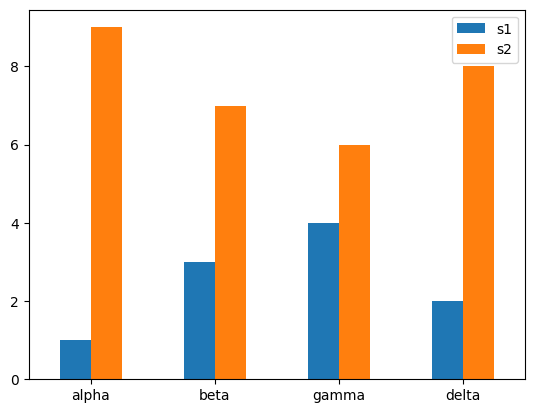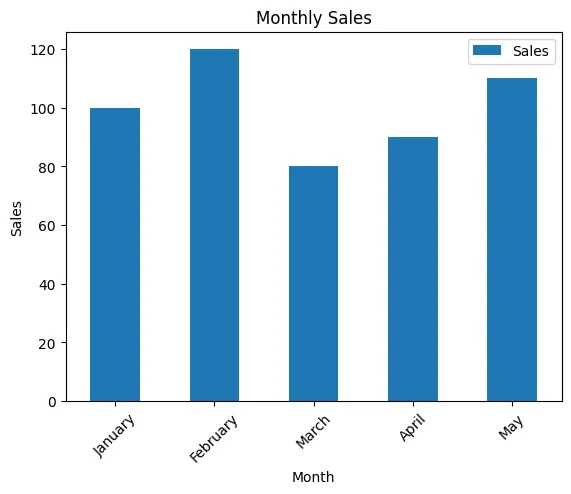To rotate the X-axis tick labels in a Pandas plot, you can use 3 different ways:

• `df.plot(kind='bar', rot=0)` - pass the parameter to plot functions applied to DataFrame
• `ax.set_xticklabels(df['Month'], rotation=45)` - by using additional method applied on plot object
• `plt.xticks(rotation=90)`
• `fig.autofmt_xdate()`

In all of them we can pass the rotation parameter to specify the angle by which you want to rotate the X labels. Depending on your plot and needs you can use them

Below you can find two examples of the most used by me:

## Steps to plot 2 variables

• Import matplotlib library
• Create sample DataFrame
• Create the axes object (optional)
• `ax = df.plot(x='Month', y='Sales', kind='bar')`
• Rotate X-axis tick labels by 45 degrees
• Add labels and title (optional)

s1 s2
variables
alpha 1 9
beta 3 7
gamma 4 6
delta 2 8

## Example 1 - `rot=0`

``````import matplotlib
import matplotlib.pyplot as plt
import pandas as pd
data = {'variables':["alpha","beta","gamma","delta"],
's1':[1,3,4,2],
's2':[9,7,6,8]}
df = pd.DataFrame(data)

df.set_index(["variables"],inplace=True)
df.plot(kind='bar', rot=0)
plt.xlabel("")
plt.show()
``````

## Output 1## Example 2 - `set_xticklabels`

``````import pandas as pd
import matplotlib.pyplot as plt

# Sample data
data = {'Month': ['January', 'February', 'March', 'April', 'May'],
'Sales': [100, 120, 80, 90, 110]}

df = pd.DataFrame(data)

# Create a plot
ax = df.plot(x='Month', y='Sales', kind='bar')

# Rotate X-axis tick labels by 45 degrees
ax.set_xticklabels(df['Month'], rotation=45)